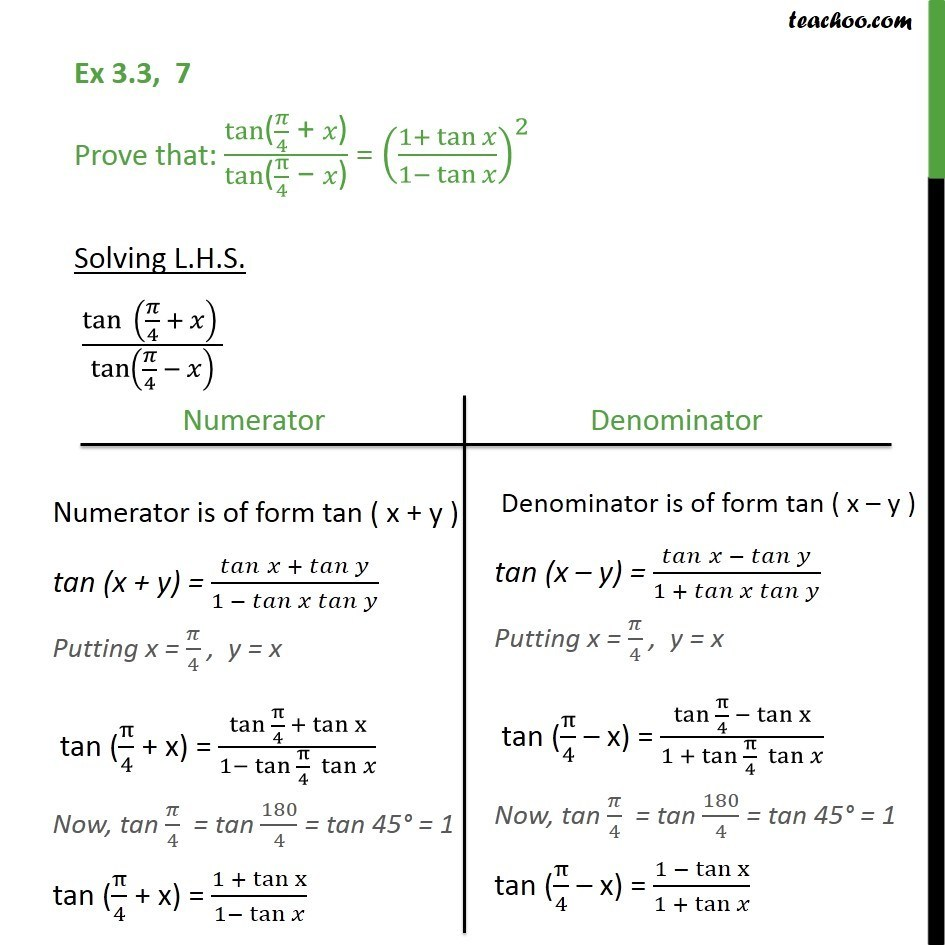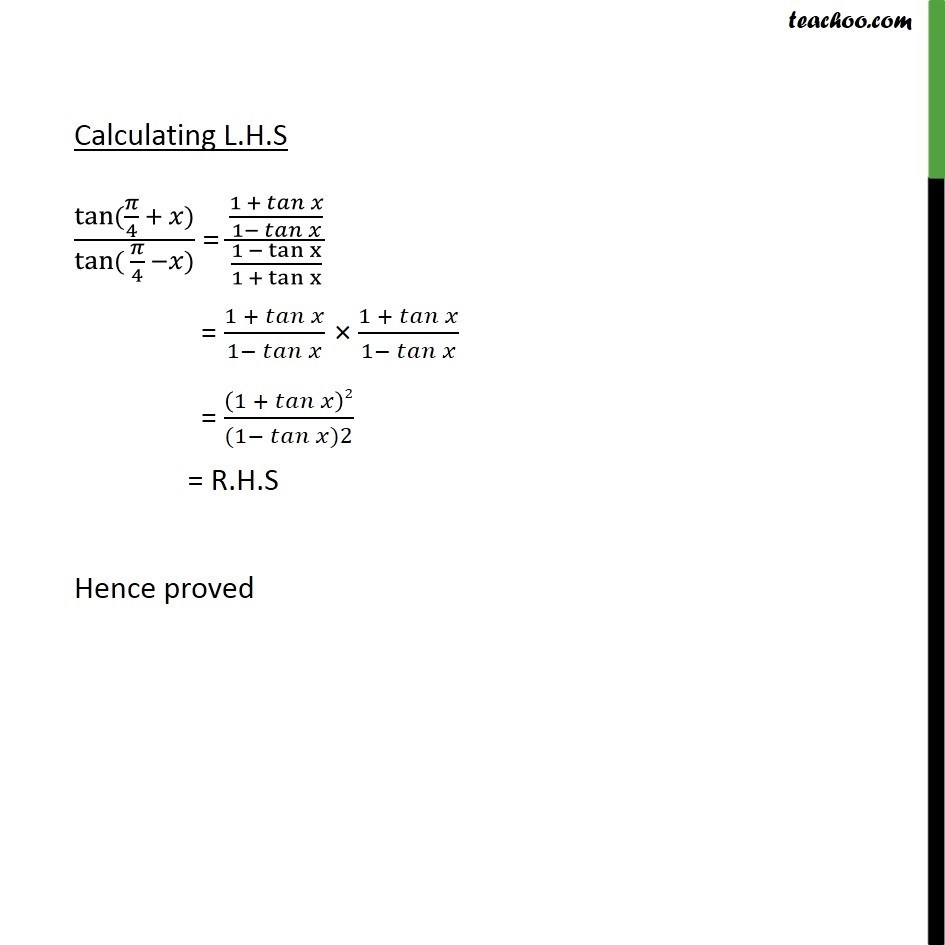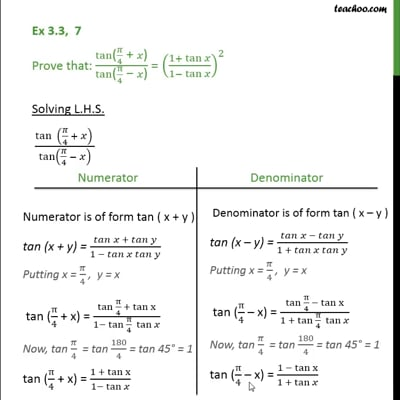(x + y) formula

Chapter 3 Class 11 Trigonometric Functions
Concept wiseThis video is only available for Teachoo black users

Introducing your new favourite teacher - Teachoo Black, at only ₹83 per month

### Transcript

Ex 3.3, 7 Prove that: (tan"(" 𝜋/4 " + " 𝑥")" )/(tan"(" π/4 " − " 𝑥")" ) = ((1+ tan" " 𝑥)/(1− tan" " 𝑥))^2 Solving L.H.S. (tan⁡ (𝜋/4 + 𝑥) )/tan⁡(𝜋/4 − 𝑥) Calculating L.H.S tan⁡〖(𝜋/4 + 𝑥)〗/tan⁡〖( 𝜋/4 −𝑥)〗 = ((1 + 𝑡𝑎𝑛⁡𝑥)/(1− 𝑡𝑎𝑛⁡𝑥 ))/((1 − tan⁡x)/(1 + tan⁡x )) = (1 + 𝑡𝑎𝑛⁡𝑥)/(1− 𝑡𝑎𝑛⁡𝑥 ) × (1 + 𝑡𝑎𝑛⁡𝑥)/(1− 𝑡𝑎𝑛⁡𝑥 ) = (1 + 𝑡𝑎𝑛⁡𝑥 )2/((1− 𝑡𝑎𝑛⁡〖𝑥)2〗 ) = R.H.S Hence proved